# AP Calculus AB : How to graph functions of points of inflection

## Example Questions

### Example Question #1 : How To Graph Functions Of Points Of Inflection

Which of the following functions has exactly one point of inflection?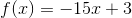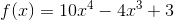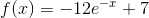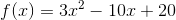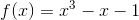Explanation:

For any function, a point of inflection occurs when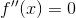.  For a function to have exactly one point of inflection, it has to be of third order (i.e. have an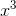term as its highest order term).  The functiononly has one point of inflection when.

A linear equation does not have any points of inflection, nor does a quadratic equation or exponential function.

A function with an order higher than three has multiple points of inflection.

### All AP Calculus AB Resources